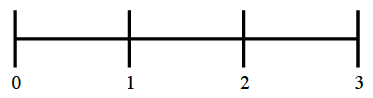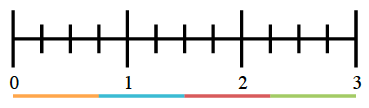### Home > CC1MN > Chapter 8 > Lesson 8.2.3 > Problem8-99

8-99.

Show how to divide $3$ pieces of licorice among $4$ people. How much does each person get?

First, draw a diagram to represent $3$ pieces of licorice.Then divide each of the three ''licorice'' into $4$ pieces to represent the $4$ people sharing the licorice.
Designate which portion is for which person.$\text{Each person gets }\frac{3}{4}\text{ of a piece of licorice.}$